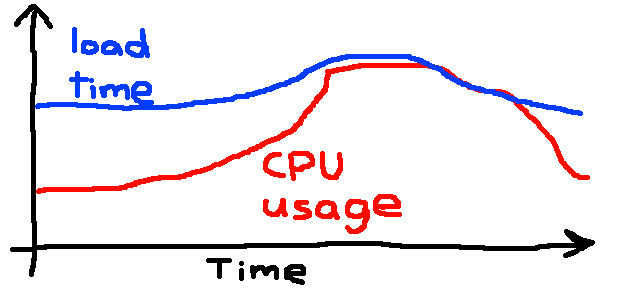# Pulling data from OpenTSDB into R

Sometimes you want to jam data from OpenTSDB into R, so you can do neat things like parameterize it by time. Well, I wrote an R function that does just that!

Here’s a usage example:

`read.opentsdb("http://localhost:44242",    metrics=c("sum:dummy.linear","sum:dummy.random.unif{blah=*}"),    start="4h-ago")`

I considered turning this into a CRAN package, but then I was like “naw.” There’s not very much to it, and writing CRAN packages seems like a big pain. Correct me if I’m wrong.

Anyway, enjoy!

# Parameterizing metrics by time through Graphite abuse

Everybody has a metric-spewing system, like StatsD, and a graphing system, like Graphite. Graphite makes time-series plots. Time-series plots are great! But they’re not everything.

I like to know how my system responds to a given stimulus. I like to characterize my product by asking questions like:

• When CPU usage rises, what happens to page load time?
• How many concurrent users does it take before we start seeing decreased clicks?
• What’s the relationship between cache hit rate and conversion rate?

In each of these cases, we’re comparing (as Theo Schlossnagle is so fond of saying) a system metric to a business metric. A system metric is something like CPU usage, or logged-in users, or cache hit rate. A business metric is something that relates more directly to your bottom-line, like page load time, click rate, or conversion rate.

Time series plots aren’t great at answering these kinds of questions. Take the first question for example: “When CPU usage rises, what happens to page load time?” Sure, you can use Graphite to superimpose the day’s load time plot over the CPU usage plot, like so:From this chart you can see that CPU usage and page load time do both tend to be higher in the afternoon. But you’re only looking at one day’s worth of data, and you don’t know:

• Whether this relationship holds on other days
• How strongly correlated the two quantities are
• What happens at lower/higher CPU usages than were observed today

To answer questions like these, what you want is a plot of page load time versus CPU usage, with time parameterized away. That is, for each moment in time, you want to plot a point for that moment. Like so:This scatterplot tells you a lot more about your system’s characteristic response to rising CPU usage. You can easily see:

• As CPU usage gets higher, page load time generally increases, but not linearly.
• For a given CPU usage, there’s a line (yellow) below which page load time will not go.
• Above a certain CPU usage (red), the relationship between load time and CPU usage becomes less strong (as evidenced by the spreading-out of the band of data points toward the right-hand side of the plot)

Time-parameterized plots like these are a great way to get to know your system. If you make an architectural change and you find that the shape of this plot has changed significantly, then you can learn a lot about the effect your change had.

But sadly, I haven’t been able to find an open-source tool that makes these plots easy to generate. So I’ll show you one somewhat ugly, but still not too time-consuming, method I devised. The gist is this:

1. Configure a graphite instance to store every data point for the metrics in which you’re interested.
2. Run a script to download the data and parameterize it with respect to time.
3. Mess with it in R

# Configure Graphite

The first thing we’re going to do is set up Graphite to store high-resolution data for the metrics we want to plot, going back 30 days.

“But Dan! Why do you need Graphite? Couldn’t you just have statsd write these metrics out to MongoDB or write a custom backend to print them to a flat file?”

Sure I could, hypothetical question lady, but one nice thing about Graphite is its suite of built-in data transformation functions. If I wanted, for example, to make a parameterized plot of the sum of some metric gathered from multiple sources, I could just use Graphite’s SUM() function, rather than having to go and find the matching data points and add them all up myself.

I’m going to assume you already have a Graphite instance set up. If I were doing this for real in production, I’d use a separate Graphite instance. But I set up a fresh disposable one for the purposes of this post, and put the following in `/opt/graphite/conf/storage-schemas.conf`:

```[keep_nconn]
pattern = ^stats\.gauges\.nconn\$
retentions = 10s:30d

[keep_resptime]
pattern = ^stats\.timers\.resptime\..*
retentions = 10s:30d

[default_blackhole]
pattern = .*
retentions = 10s:10s```

This basically says: keep every data point for 30 days for `stats.gauges.nconn` (number of concurrent connections) and `stats.timers.resptime` (response times of API requests), and discard everything else.

# Get parametric data

I wrote a script to print out a table of data points parameterized by time. Here it is: https://gist.github.com/danslimmon/5320247

# Play with it in R

Now we can load this data into R:

`data <- read.table("/path/to/output.tsv")`

We can get a scatterplot immediately:

`plot(data\$resptime_p90 ~ data\$nconn)`There’s a lot of black here, which may be hiding some behavior. Maybe we’ll get a clearer picture by looking at a histogram of the data from each quartile (of nconn):

```# Split the graphing area into four sections
par(mfrow=c(2,2))
# Get quantile values
quants <- quantile(data\$nconn, c(.25,.5,.75))
# Draw the histograms
hist(data\$resptime_90p[data\$nconn <= quants], xlim=c(0, 200), ylim=c(0,70000), breaks=seq(0,2000,20), main="Q1", xlab="resptime_90p")
hist(data\$resptime_90p[data\$nconn > quants & data\$nconn <= quants], xlim=c(0, 200), ylim=c(0,70000), breaks=seq(0,2000,20),  main="Q2", xlab="resptime_90p")
hist(data\$resptime_90p[data\$nconn > quants & data\$nconn <= quants], xlim=c(0, 200), ylim=c(0,70000), breaks=seq(0,2000,20),  main="Q3", xlab="resptime_90p")
hist(data\$resptime_90p[data\$nconn >= quants], xlim=c(0, 200), ylim=c(0,70000), breaks=seq(0,2000,20), main="Q4", xlab="resptime_90p")```

Which comes out like this:This shows us pretty intuitively how response time splays out as the number of concurrent connections rises.